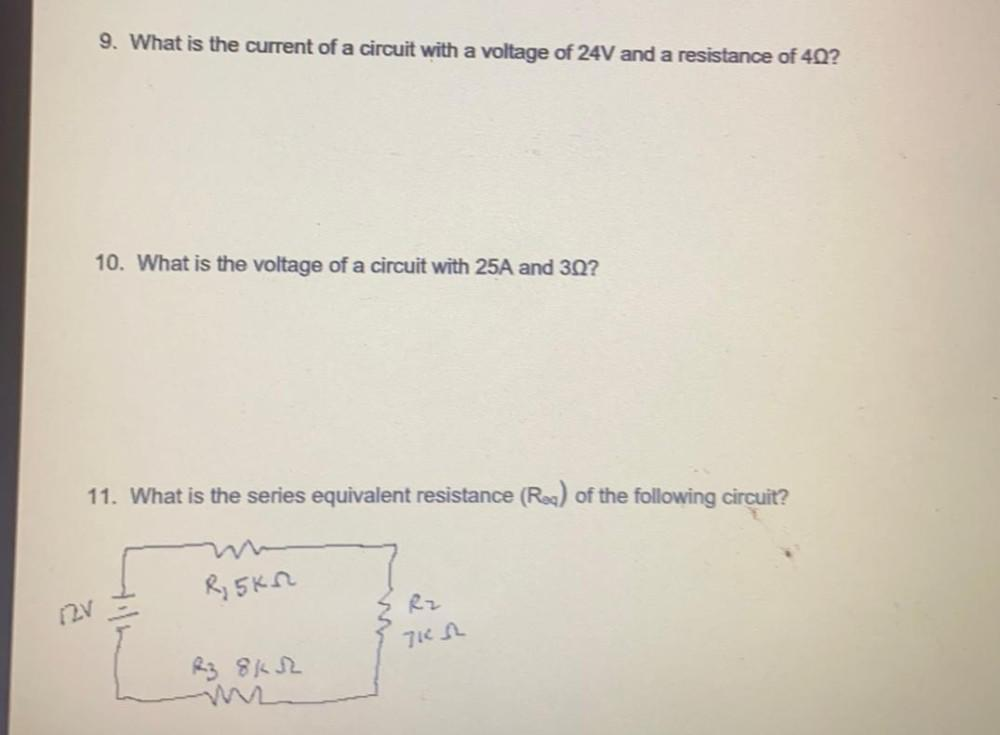Question:

# 9. What is the current of a circuit with a voltage of 24V and a resistance of 40? 10. What is the voltage of a circuit with 25A9. What is the current of a circuit with a voltage of 24V and a resistance of 40? 10. What is the voltage of a circuit with 25A and 3Q? 11. What is the series equivalent resistance (Roa) of the following circuit? R, 5KM Rz 12v 71n kez 8KR